AnyLogic

# Output

The Output element is used for storing a single scalar data value and displaying it after (or during) the model run. Examples of such values include important quantitative indicators (mean time in system, profit, expenses, customer satisfaction level, etc.), which provide an “at a glance” view of the simulation results.

The Output elements are primarily used for displaying values in the dedicated Outputs section of the experiment screen in AnyLogic Cloud. To add outputs to the cloud version of your model — as well as other elements of the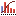Analysis palette — use the Run Configuration editor.

You define an output by providing its Value in the form of an arbitrary Java expression. Commonly, this expression is evaluated at the end of the simulation experiment. If needed, you can evaluate it at the predefined moment in time or manually by calling the update_<output name>() function from any place of the model. For more information on programmatically accessing various parts of the model, which you can use in an expression, refer to the Where am I and how do I get to…? section.

Similarly to a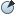Parameter, an output can be of any Java primitive type (int, double, boolean, or String). Unlike a parameter, an output cannot contain values of the Object class. You can also set the output to one of the measurable types (i.e., time, rate, length, speed, acceleration, area, amount or flow rate) and select the corresponding measurement units for it. For example, if Length is selected as the Output’s Type, length units (inch, mile, meter, etc.) will be available for selection.

If a measurable type is selected for the output, its values data type is implicitly set to double.

For example, you have a pedestrian model involving a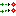Service with Lines element and you would like to view the current length of the queue line during the model run. To implement this, you can add an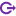Output element and set its Value to queueName.size(), which will return the length of the queue. This value should be updated each time a pedestrian enters or exits the queue. For this reason, you should call the update_<output name>() function in the On enter queue and the On exit queue actions of the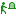PedService flowchart block. If in some other case you need to update the output’s value periodically, you can add a Timeout Triggered Event to your model, set its Mode to Cyclic and provide the update_<output name>() function call in the event’s Action field.

To create an output

1. Drag theOutput element from theAnalysis palette into the graphical editor.
2. Go to the Properties view.
3. Type the name of the Output in the Name edit box. The name is used to identify and access the Output.
4. If your Output defines one of the following: time, speed, length, acceleration, rate, or area, you should specify this explicitly by choosing the corresponding Type for this parameter. Then choose the Unit from the drop-down list below.
5. Otherwise, specify the Java type for the Output. You can choose one of the most used Java types (int, double, boolean, String) by selecting the corresponding option from the Type drop-down list.
6. Specify the output’s value in the Value edit box in the form of an arbitrary Java expression. The value of the output can be changed later during the model simulation by using the update_<output name>(<new value>) function.
7. Finally, choose, when the output value should be calculated by selecting one of the available Calculated options. Value can be calculated either automatically (at the specified moment in time or at the end of the simulation experiment) or manually on calling the update_<output name>() function.

## Properties

General

Name — The name of the output. The name is used to identify and access the output.

Ignore — If selected, the output is excluded from the model.

Visible — If selected, the output is visible on the presentation at runtime.

Show name — If selected, the name of the output is displayed on the presentation diagram.

Type — The type of the output. Choose a measurable type (Time, Speed, Length, etc.) or one of the Java primitive types (int, double, boolean, String) using the corresponding option from the list. Note that if a measurable type is selected for the Output, its values data type is implicitly set to double.

Unit — [Visible if Type is set to one of the measurable types (Time, Speed, Length, etc.)] The measurement units of the output’s value. The set of available units depends on the currently selected Type. For example, if Length is set as the Output type, length units (inch, mile, meter, etc.) will be available for selection.

Value — The value of the output. Here you provide a Java expression that will be used for calculating the value. The provided expression will be evaluated according to the Calculated option. If a value is not specified, Java rules apply, for example a parameter of type double is set to 0, a parameter of type boolean is false, a parameter of type String is null.

Calculated — The time at which the output’s value is calculated. In most cases, the value of the output is supposed to be calculated on simulation end.

On simulation end — the value is calculated after the simulation is finished.
At model time — the value is calculated after the certain number of time units pass since the simulation start. The number of time units is specified using the Update time (absolute) controls below.
At calendar date — the value is calculated at the specified model date, which is specified using the Update date controls below.
User control (call update()) — the value is calculated when the update_<output name>() function is called. If no arguments are passed to the function, the expression specified in the Value field will be evaluated. If an argument is passed (i.e., update_<output name>(<new value>)), it will be evaluated and used as the output’s value.

Update time (absolute) — [Visible if Calculated is set to At model time] the time period since the simulation start, after which the output’s value will be calculated.

Update date — [Visible if Calculated is set to At calendar date] the model date, at which the output’s value will be calculated.